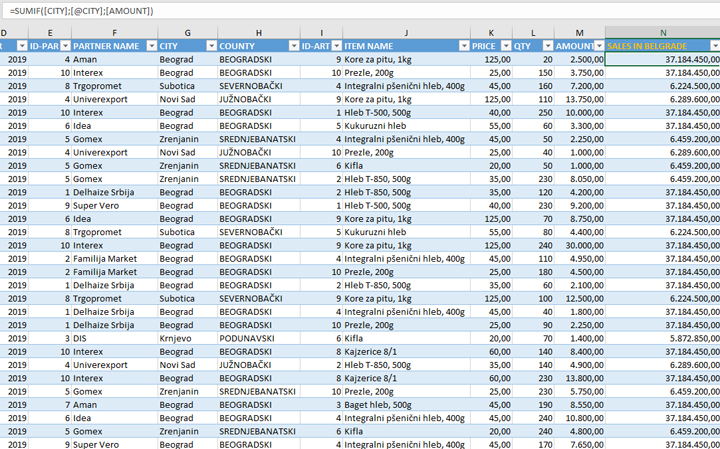# Current row

Structured tables (Tables) make it much easier for us to work with data. When we convert a range of data into a table and then give it a suitable name, we can easily access each column by specifying its name or a combination of table and column names. By entering a formula in the first cell, it is automatically updated in remaining cells within the column. And what if we don’t want that? What should we do if we need to use the current row value?

In the example, you can see a table that contains the sales transactions of one bakery. If it is necessary to calculate the coefficient by which we would compare the sales of the current product and all those sold in Belgrade (Beograd), we should first write a formula that calculates the total sales in Belgrade. We will do this with the help of the SUMIF function, where we list the column names as arguments. But how to refer to the value of the city in the current row? By listing the @ sign in front of her name! In the formula, it looks like this:

=SUMIF([CITY],[@CITY],[AMOUNT])

The first parameter of the SUMIF function is the column where we are searching values. The second parameter is what we’re searching for i.e. the value of the current order, which is “Belgrade”. The third parameter is the column to be summarized (AMOUNT). As a result, we get the total sales in the city of Belgrade within entire table. To calculate the share of sales, we only need to divide the sales of the current product by the previously calculated total sales in Belgrade.